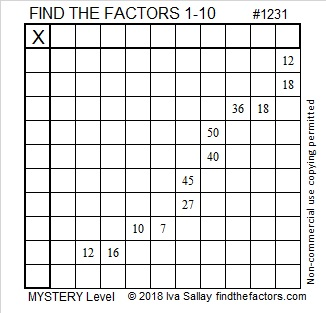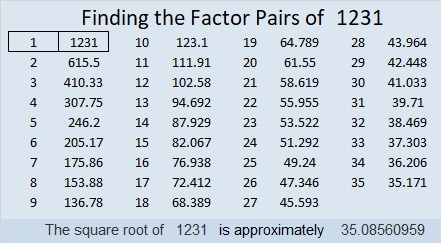# 1231 Mystery Level Puzzle

For almost all the sets of clues in this puzzle, there is more than one permissible common factor. That makes the puzzle a little tricky, but with care, you can still solve it using logic and your knowledge of the basic 10×10 multiplication table. Good luck!Print the puzzles or type the solution in this excel file: 10-factors-1221-1231

Now I’ll tell you a little bit about the number 1231:

• 1231 is a prime number.
• Prime factorization: 1231 is prime.
• The exponent of prime number 1231 is 1. Adding 1 to that exponent we get (1 + 1) = 2. Therefore 1231 has exactly 2 factors.
• Factors of 1231: 1, 1231
• Factor pairs: 1231 = 1 × 1231
• 1231 has no square factors that allow its square root to be simplified. √1231 ≈ 35.08561

How do we know that 1231 is a prime number? If 1231 were not a prime number, then it would be divisible by at least one prime number less than or equal to √1231 ≈ 35.1. Since 1231 cannot be divided evenly by 2, 3, 5, 7, 11, 13, 17, 19, 23, 29 or 31, we know that 1231 is a prime number.1231 is a palindrome in a couple of bases:
It’s A1A in BASE 11 because 10(11²) + 1(11) + 10(1) = 1231, and
it’s 1B1 in BASE 30 because 1(30²) + 11(30) + 1(1) = 1231

This site uses Akismet to reduce spam. Learn how your comment data is processed.